## what must be added to x3-3×2-12x+19 so that the result is exactly divisible by x2+x-6?

Question

what must be added to x3-3×2-12x+19 so that the result is exactly divisible by x2+x-6?

in progress 0
8 months 2021-07-18T05:34:53+00:00 1 Answers 8 views 0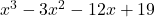is divisible by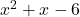when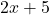is added to it.

Step-by-step explanation:

Let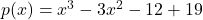&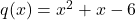By division algorithm, when p(x) is divided by q(x), the remainder is a linear expression in x

So, let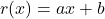is added to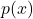so that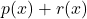is divisible by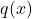Let,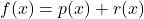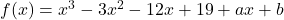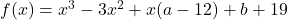We have,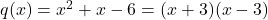Clearly,is divisible by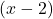and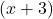{andare factors of}

We have,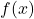is divisible byandare factors ofFrom factors theorem,

Ifandare factors ofthen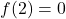and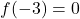respectively,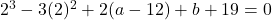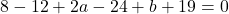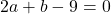Similarly,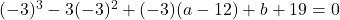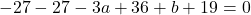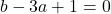Subtract (1) from (2)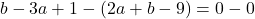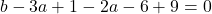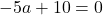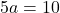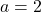Putin equation 2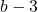×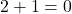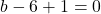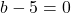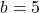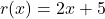Hence,is divisible bywhenis added to it.

Hope this helps…